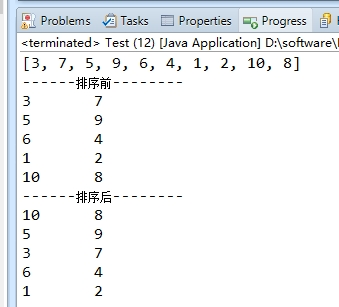1、现在随机生成1-10这10个数，不重复的。

2、现在有业务需求：计算每组数据的两数之和，

3个回答

`````` package baokexiao.test;

import java.util.Arrays;
import java.util.Random;

public class Test {
public static void main(String[] args) {
int [] array = getRandomArray();
System.out.println(Arrays.toString(array));
int [][] arrayGruop = splitArray(array);
System.out.println("------排序前--------");
printArrayGroup(arrayGruop);
sortArrayGroup(arrayGruop);
System.out.println("------排序后--------");
printArrayGroup(arrayGruop);

}

//数组排序
public static void sortArrayGroup(int [][] arrayGruop){
//冒泡排序
for (int i = 0; i < arrayGruop.length -1; i++){    //最多做n-1趟排序
for(int j = 0 ;j < arrayGruop.length - i - 1; j++){    //对当前无序区间score[0......length-i-1]进行排序(j的范围很关键，这个范围是在逐步缩小的)
if(compareArray(arrayGruop[j],arrayGruop[j + 1]) == -1){    //把小的值交换到后面
int [] temp = arrayGruop[j];
arrayGruop[j] = arrayGruop[j + 1];
arrayGruop[j + 1] = temp;
}
}
}
}

//数组比较大小
public static int compareArray(int [] array1,int [] array2){
int sum1 = getArraySum(array1);
int sum2 = getArraySum(array2);
if(sum1 > sum2) return 1;
if(sum2 < sum2) return -1;
int max1 = getArrayMaxVal(array1);
int max2 = getArrayMaxVal(array2);
if(max1 > max2) return 1;
if(max1 < max2) return -1;
throw new RuntimeException("can not compareArray,array1:"+Arrays.toString(array1)+" array2:"+Arrays.toString(array2));
}

//打印数组
public static void printArrayGroup(int [][] array){
for (int i = 0; i < array.length; i++) {
for (int j = 0; j < array[i].length; j++) {
System.out.print(array[i][j]+"\t");
}
System.out.println();
}
}

//拆分数组
public static int[][] splitArray(int [] array){
int groupSize = array.length/2;
int [][] result = new int[groupSize];
int foot = 0 ;
for (int i = 0; i < groupSize; i++) {
int [] tmp = new int;
tmp = array[foot++];
tmp = array[foot++];
result[i] = tmp;
}
return result;
}

//求数组中的最大值
public static int getArrayMaxVal(int [] array){
int max = array;
for (int i = 1; i < array.length; i++) {
if(array[i] > max) max = array[i];
}
return max;
}

//求数组的和
public static int getArraySum(int [] array){
int result = 0;
for (int i = 0; i < array.length; i++) {
result += array[i];
}
return result;
}

//创建数组
public static int [] getRandomArray(){
int [] result = new int ;
Random r=new java.util.Random();
for (int i = 0; i < result.length; i++) {
int t = r.nextInt(10)+1;//[1,10)
while(checkRepeat(result,t)){
t = r.nextInt(10)+1;
}
result[i] = t;
}
return result;
}
//查看是否重复
public static boolean checkRepeat(int [] array,int t){
for (int i = 0; i < array.length; i++) {
if(t == array[i]) return true;
}
return false;
}
}

````````````
import java.util.Comparator;
import java.util.Random;
import java.util.TreeSet;

public class Test4Sort {

private TreeSet< int[]> treeSet = new TreeSet<int[]>(new Comparator<int[]>() {
@Override
public int compare(int[] o1, int[] o2) {
int sum1 = o1 + o1;
int sum2 = o2 + o2;
if(sum1 > sum2){
return 1;
}else if(sum1 == sum2){
int max1 = o1 > o1 ? o1 : o1;
int max2 = o2 > o2 ? o2 : o2;
if(max1 > max2){
return 1;
}else{
return -1;
}
}else{
return -1;
}
}
});

public static void main(String[] args) {
Test4Sort sort = new Test4Sort();

for(int i=0;i<5;i++){
int[] temp = new int;
for(int j=0;j<2;j++){
temp[j] = new Random().nextInt(10)+1;
}
}

for (int[] ints : sort.treeSet) {
System.out.print(ints+","+ints+"<");
}
}

}

``````Android比较数据大小

package w; import java.util.Scanner; //添加输入库文件包 public class ConOperation { //创建类ConOperationpublic static void main(String[] args) { // TODO 自动生成的方法存根 int a,b,c; //定义变量 System.out.
java 比较数据大小
import java.io.*;//引入需要加';' public class text2 { public static void main(String[] args) { // int a = 2; // int b = 3; // if (b%2==0) { // System.out.println("可以整除"); // }else { // System.

c++实现大小比较
c++实现大小比较.

smalldatetime类型的数据如何比较大小
smalldatetime类型的数据如何比较大小，应该不可以这样a>b(a和b是smalldatetime的变量)是吧 那该怎么比较呢

[code=VBScript]rnif cdbl(allgls)< cdbl(5) and cdbl(alllx)>cdbl(3) thenrn jg1=50+1.5*allgls*lfmrnend ifrnif cdbl(allgls)< cdbl(5) and cdbl(alllx)cdbl(3) thenrnresponse.write "hao"rn jg2=30+1.5*allgls*lfmrnend ifrnif cdbl(5)= cdbl(10) and cdbl(alllx)>cdbl(3) thenrn jg3=10+1*allgls*lfmrnend ifrnif cdbl(allgls)>= cdbl(10) and cdbl(alllx)

if ('b0'<'b-')rnprint '1'rnrnif ('b099999'<'b-99999')rnprint '1'rnrnrn为什么两个判断的结果会不一样？

RGB 数据如何量化，如何比较两个RGB数据的大小？

//在数据库中oldtime和nowtime为date类型         Date dt1 = df.parse(oldtime);    Date dt2 = df.parse(nowtime);    if (dt1.getTime()&amp;lt;dt2.getTime()) { System.out.println(“haha”); } ...

Log4j打出的时间格式是rn2006-05-18 16:27:10,125rn而我现在要比较的时间大小，例如我在命令行输入rn2006-05-18 10:10:10rn那么我就只关注2006-05-18 10:10:10rn这个时间后面的日志信息rn但是现在的问题的是java好像没有提供直接时间比较的方法rn我查拉Date和Calendar两个类，好像也没有提供类似的方法rn我所以我现在想出来的办法就是rnINFO 2006-05-18 16:27:10,125 - -->1147940830125-->UPDATED-->DN=cn=傅贵服,ou=Groups,o=非官方个,o=党群事务部,dc=gmcc,dc=netrn1147940830125这个其实就是2006-05-18 16:27:10,125的millisecond标示rn对应代码就是System. currentTimeMillis(),rn当用户在命令行输入2006-05-18 10:10:10之后rnCalendar cal = Calendar.getInstance();rncal.set(2006, 5, 18, 10,10,10);rnlong stime = cal.getTimeInMillis();　//这也会是millisecond来标示的时间rn然后就可以通过毫秒时间来进行比较拉rn感觉这实在是无奈之举，因为log4j本身已经打印了当前时间，但是我为了比较时间大小又取了一次当前时间，而且如果log4j本身取当前时间的实现就用了System. currentTimeMillis()，那我的代码可真的是冗余之至。rn请问大虾还有其他方便的比较方法吗？我是不是很土阿rn

string startdate="18:30:00";rnstring enddate="02:30:00";rnstring nowdate="20:30:00";rnDatetime dstartdate=convert.todatetime(startdate);rnrnrn如何判断nowdate 是否在 startDate和EndDate间？注意startDate 和EndDate间差了一天rnrnrn

BigDecimal如何比较大小
BigDecimal是Java里精确计算的类，下面说一下两个BigDecimal对象大小，相等的判断。         一般的对象用equals，但是BigDecimal比较特殊，举个例子： BigDecimal a=BigDecimal.valueOf(1.0); BigDecimal b=BigDecimal.valueOf(1.000);         在现实中...

tabel1下的A字段和table2下的B字段都是存的电话号码，我想比较一下这两个字段下不同的电话号码，并把他显示出来，这段程序有没有办法实现？（注：A字段和B字段是一种包含关系，也就是说A字段包含了B字段所有的号码）

a1=2004-12-24 9:30:12rna2=2004-12-24 17:00:00rnrn如何比较二者的大小

[code=Java]rn String orderdate="2012-02-27 19:21:11";rn SimpleDateFormat sdf=new SimpleDateFormat("yyyy-MM-dd HH:mm:ss");rn Date date=new Date();rn String date2=sdf.format(date);rn //orderdate 与 date2 如何进行时间比对，如何比对出哪个值要大？[/code]rnrn之前有想过转换成Int类型进行比对，但是长度太大了 ，超出了int类型的长度，哪位大哥能帮个忙么？